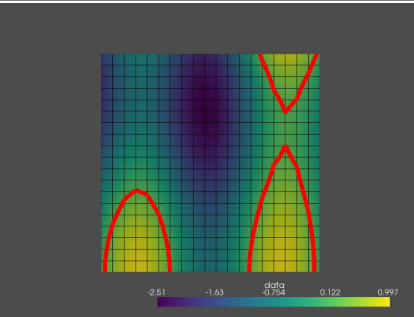# create connected contour lines from set of points

Hi I am new to Python, VTK and pyvista.

I am trying to implement from scratch (i.e., without using any library functions for contouring or interpolation ) an iso contour algorithm.

I am using pyvista to create a custom grid and render it.

With iso_contour function i am pushing (x, y, 0) points but i am not able to connect them to make an island of isocontour !!

I am here to ask help from you if you could help me achieve this using vtk or any other thing !

This is my code

``````def f(x, y):
return np.sin(10*x)+np.cos(4*y)-np.cos(3*x*y)

x = np.arange(0, 1, 0.05)
y = np.arange(0, 1, 0.05)
XX, YY = np.meshgrid(x, y)
data = f(XX, YY)

isovalue = 0.15

def iso_contour(array, target_value):
contours = []
rows, cols = array.shape

# Loop through each cell in the array
for i in range(rows-1):
for j in range(cols-1):
cell_verts = []

# Check each corner of the cell
for corner in [(i,j), (i+1,j), (i+1,j+1), (i,j+1)]:
x, y = corner
cell_verts.append((x, y, array[x,y]))

# Check each edge of the cell
for k in range(4):
p1, p2 = cell_verts[k], cell_verts[(k+1)%4]
if (p1 >= target_value) != (p2 >= target_value):
# Calculate intersection point
t = (target_value - p1) / (p2 - p1)
x, y = p1[:2] + t * (np.array(p2[:2]) - np.array(p1[:2]))
contours.append((y, x, 0.0))

return contours

contour_points = iso_contour(data, isovalue)
pl = pv.Plotter()
for i in range(len(contour_points)-1):
line = pv.Line(contour_points[i], contour_points[i+1])